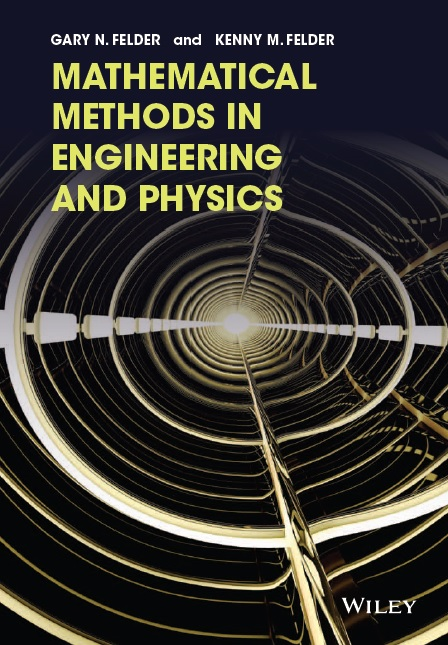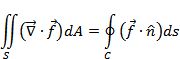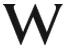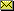# Mathematical Methods in Engineering and Physics:Errata

## by Gary N. Felder and Kenny M. Felder

Below are the errors we are aware of in the text. Of course if you've found any others we want to hear about them. Please email us at the link at the bottom of the page.

• Page xiii: "the most minimal treatment possibly" should read "the most minimal treatment possible."
• Page 20: Problem 1.43 should have a / in both the differential equation and the solution:
(1-2xy) / (x^2+1) and (C+x) / (x^2+1)

(In the solution manual Part b of this problem is written as if the given solution were a product, but it should be written for a quotient as indicated here.)
• Page 116: Problems 3.47 and 3.48 refer to "magnitude" when they should say "modulus." Problem 3.48 also says "angle" when it should say "phase."
• Page 134: Part g of Problem 3.117 should have a sum going from n=1 to N instead of to 5.
• Page 174: The paragraph toward the bottom of the page (beginning "Recall that a critical point") has two minor errors. In the equation 40-L2-12LH=0, substitute 3L2 for the middle term; in the phrase "Solving these two equations simultaneous" substitute "simultaneously" for the fifth word.
• Page 195: Problem 5.2 Part (a) says "for the first integral" when it should say "for the first interval."
• Page 207: The next-to-last sentence in the "Double Integral" example ("The constant sigma also appeared...the problem was using.") came from a completely different scenario, and makes no sense in this one. Ignore it and the rest of the example makes sense.
• Page 233: The final solution to the "Example" gives the spherical coordinates of points A, B, and C in the order (r,phi,theta) rather than the correct order (r,theta,phi). Also, the theta coordinates for Points B and C should be tan-1(sqrt(2)) and pi-tan-1(sqrt(2)).
• Page 245: Problem 5.205 says (vector) f(x,y) where it should say (vector) f(x,y,z).
• Page 246: Problem 5.221 should say that you can use the approximation that gravity points along the z axis.
• Pages 250-251: In the example we write the equation for y with cos u when it should say sin u, once at the bottom of Page 250 and again at the top of Page 251.
• Page 254: "How much ... will each of the other two buckets accumulated" should say "have accumulated."
• Page 285: In Problem 6.35 the phrase "Icelandic population" should have a comma after it.
• Page 299: Equation 6.5.1 shows e times the column (a,c) plus f times the column (b,d). The second term should be g times (b,d), not f.
• Page 300: In the final paragraph the sentence "The mass of the slice is the total mass of the cone divided by the fraction of the cone's volume taken up by the slice." should say "multiplied by" instead of "divided by."
• Page 307: The upper-right-hand corner of X-1 should be -17/18, not 17/18 as written. This error appears in two separate equations, but the final result (molecules of methane and butane) is correct.
• Page 314: The box in the middle of the page is correct. However, in the sentence immediately below that box, substitute "linearly dependent" for the incorrect phrase "linearly independent."
• Page 317: The sentence "Ask a computer for the determinant of a 2x3 matrix you and should get an error" should say "and you" instead of "you and."
• Page 324: Problem 6.161 works as written if you treat it as pure math, but cannot correspond to a physical three-spring scenario. It makes a useful exercise for students to explore why this is so.
• Page 373: Near the top of the page, the last entries under "Real matrices" and "Complex matrices" both say that the eigenvectors must have modulus 1. They should say that about the eigenvalues, not eigenvectors.
• Online Section 7.5, Page 21: Problem 7.106 says "the bottom edge has F=0" when it should say "the left edge has F=0."
• Pages 388-389: The Earth-moon example has two problems. First, it assigns the Earth a mass six times that of the moon. This makes for a good example, but we should have clearly stated (at least in a footnote) that the actual ratio is more like 81 to 1. Second, Equation 8.3.1 does not have a maximum of -0.3 (as the text says) or -0.03 (as the graph says), but of -0.03GM.
• Page 401: Problem 8.63 ends with the phrase "throughout the room." That should be replaced with "on the surface of the table." It also would be a clearer problem if it specified the dimensions of the table, so at the end of the problem it should say "Assume the surface of the table extends from (-2,-2) to (2,7)." If a student hadn't seen this correction and chose their own dimensions that would be fine as long as it included the two light sources.
• Page 437: Problem 8.169 Part (e) uses the phrase "total contribution go the curl" instead of "total contribution to the curl" as it should.
• Online Section 8.12, Page 1: Problem 8.200 should have "v=" at the start.
• Page 464: In the paragraph just above the Example, change 812 Kz to 882 Hz and change "slightly higher than G5" to A5.
• Page 465: In Problem 9.42 Part (d), change "next largest period" to "next smallest period."
• Page 466: The block of problems that begins "For Problems 9.48-9.50 ..." should instead begin "For Problems 9.48-9.51 ..."
• Page 476: The last sentence would be clearer if it said "cos(3p)" instead of "cos(3x)."
• Page 478: The last formula in the "Very Important Fact" box should have e^(-i p x0) instead of e^(i p x0). (This same mistake appears in Appendix G on p. 781.)
• Page 482: The instructions for Problems 9.79-9.83 should say "Label the p-values" (not "the x-values") on the horizontal axis.
• Online Section 10.3, Page 10: In Problem 10.61 ("Inflationary Cosmology") the equation is missing phidot (a phi with a dot over it) in front of the square root.
• Online Section 10.6, Page 27: The "Check Yourself" for Part 1 of Discovery Exercise 10.6.1 is #64, not #66. Note that this is correct if you download the standalone Discovery Exercise, but incorrect if you download the entire section.
• Page 563: Problem 11.52 Part (c) says "you found Part (b)." It should say "you found in Part (b)."
• Page 602: In Equation 11.6.15 the argument to J1 should have a rho0, not a rho.
• Page 608: Under the heading "Separating the Variables" it says that you will derive Equations 11.7.1 and 11.7.2 in Problem 11.157. In fact that problem solves Equation 11.7.1 rather than deriving it.
• Pages 614-615: Problem 11.159 says that the explanation derived the formula for the gravitational potential inside a spherical shell with specified boundary conditions. In fact the explanation referred to electric potential, not gravitational potential, but the result is the same.Click here for the home page for the book, http://www.felderbooks.com/mathmethods.Click here for the table of contents for the book, http://www.felderbooks.com/mathmethods/contents/.Click here for a link to Wiley's site where you can request a free evaluation copy of the book.If you have questions, we would love to hear from you. Click here to email the authors at GFelder@Smith.edu.